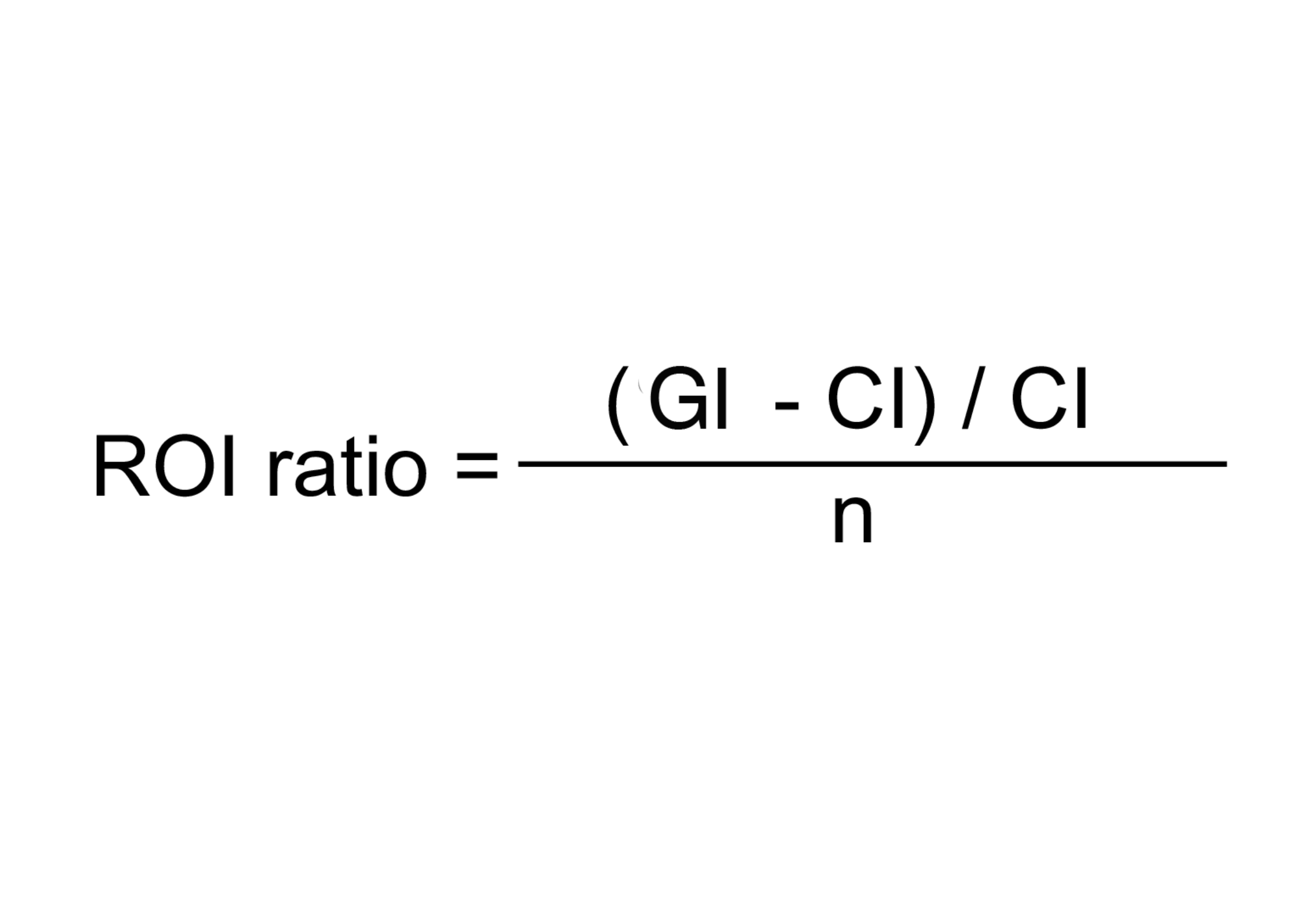﻿ ROI ratio: - on line calculation, formula - FORMIAX

# ROI ratioCI = cost of investment

GI = gain from investment

n = počet rokov

WHAT IS IT ?
Return on Investment (ROI) is a performance measure, used to evaluate the efficiency of an investment or compare the efficiency of a number of different investments. ROI measures the amount of return on an investment, relative to the investment’s cost. To calculate ROI, the benefit (or return) of an investment is divided by the cost of the investment. The result is expressed as a percentage or a ratio.

CALCULATION:

Enter returned amonut - market shares price

Enter investment amount

Enter investment time in years

YOU MIGHT BE INTERESTED: